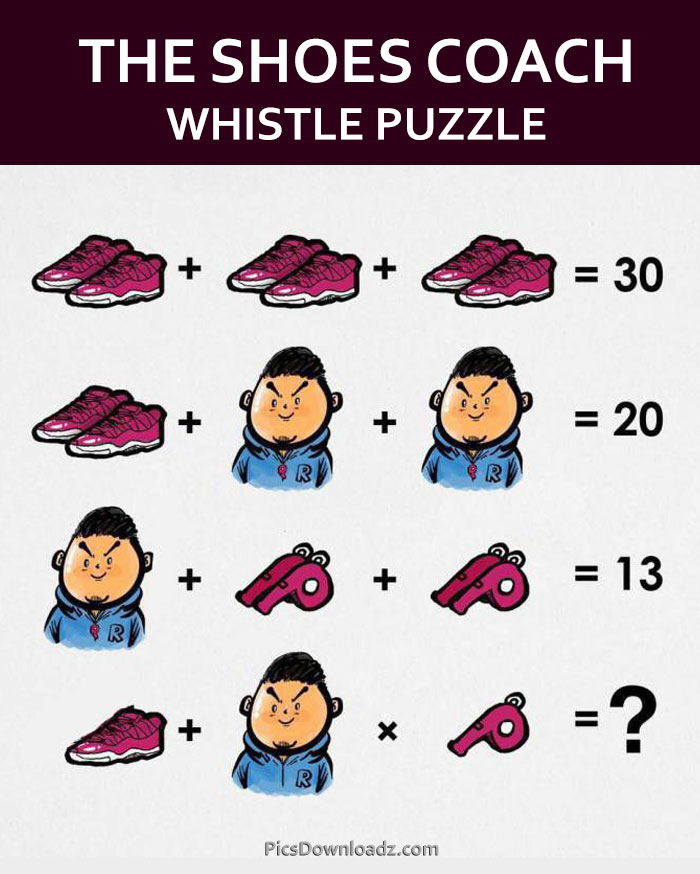This post may contain affiliate links. This means if you click on the link and purchase the item, We will receive an affiliate commission at no extra cost to you. See Our Affiliate Policy for more info.

The Shoes, Coach and Whistle Puzzle – Viral Whatsapp Puzzle (With Answer)

6
14642

How does one solve this pair of shoes, coach and whistle puzzle? This Math Puzzle From China Stumps Internet. Can you solve this viral WhatsApp brainteasers math puzzle?

Here is another unique and fun math puzzle for you. This math problem is getting viral on Whatsapp for a reason. Let’s find out what makes this puzzle so interesting and confusing.

Find out the correct value of shoes, coach, and whistle and then solve the last equation of the puzzle. The puzzle question is as follow:

⇒ Shoes + Shoes + Shoes = 30

⇒ Shoes + Coach + Coach = 20

⇒ Coach + Whistle + Whistle = 13

⇒ Shoes + Coach × Whistle = ??

(Check image for details)Interesting! isn’t it? Got the answer? Share your answer below, the comment section is all yours. And for the answer and solution… keep scrolling.

.

A

N

S

W

E

R

.

For this Puzzle, the Correct answer is 11. Check the solution:

Equation 1:

⇒ Shoes + Shoes + Shoes = 30

⇒ 3 Shoes = 30

⇒ Shoes = 30/3 = 10

So, The Pair of Shoes = 10

Therefore the Single Shoe = 5

Equation 2:

⇒ Shoes + Coach(with a Whistle) + Coach(with a Whistle) = 20

Put the value of Pair of Shoes = 10 (from first equation). Now above equation become:

⇒ 10 + Coach(with a Whistle) + Coach(with a Whistle) = 20

⇒ 10 + 2 Coach(with a Whistle) = 20

⇒ 2 Coach(with a Whistle) = 20 – 10

⇒ 2 Coach(with a Whistle) = 10

⇒ Coach(with a Whistle) = 10/2 = 5

So we get, Coach(with a Whistle) = 5

Set it aside.

Equation 3:

⇒ Coach(with a Whistle) + 2 Whistle + 2 Whistle = 13

⇒ Coach(with a Whistle) + 4 Whistle = 13

We got the value of Coach(with a Whistle) = 5 (from 2nd equation). Now put it in above equation;

⇒ 5 + 4 Whistle = 13

⇒ 4 Whistle = 13 – 5

⇒ 4 Whistle = 8

⇒ Whistle = 8/4 = 2

⇒ Whistle = 2

Last Equation:

⇒ Shoes + Coach × Whistle = ??

The tricky part is two of the pictures in the last line have slight differences from the previous equations. Compare these pictures from previous equations and then you will understand the puzzle correctly.

I.e.

• Shoes(Pair) from eq.1  Vs  Shoe(Single) In last equation
• Coach(with a Whistle) in above eq.  Vs  Coach(without Whistle) In last equation
• And of course, Single Whistle in the last equation Vs Double Whistle in 3rd eq.

Let’s rewrite the last equation:

⇒ Shoe (Single) + Coach(without Whistle) × Single Whistle = ??

We know:

• Shoes (Pair) = 10, Therefore Shoe (Single) = 5
• Single Whistle = 2,
• Coach(with a Whistle) = 5, Therefore Coach(without Whistle) = 5 – 2 = 3

Now put these values in the last equation;

⇒ Shoe (Single) + Coach(without Whistle) × Single Whistle = ??

⇒ 5 + 3 × 2 = ?? (Now apply BODMAS, Do multiplaction first)

⇒ 5 + 6 = ??

⇒ 5 + 6 = 11 Answer

If you assumed Coach = 5 immediately, you’ll get it wrong.

Note: This answer is for Single Shoe in the last equation of this puzzle. If you have Pair of Shoes in the last equation then you will get 16 as an answer, which is also correct.

Like our Page:

Search items:

shoe man puzzle, Genius Math Puzzle Images, shoes+shoes+shoes=30 whatsapp puzzle, Viral Facebook math Puzzles, Tricky Math Puzzle Image, Logical Puzzle Question, Confusing Math Problem, Puzzles for Whatsapp, Only for Genius Puzzles, Viral Whatsapp Math Puzzles, Fun Math Question, Logical Brainteaser Puzzles.

1.Poonam

25

2.Charleen

It’s 30

3.Yahya

Man(5) x Whistle(4) = 20
20 + Shoe(10) = 30

4.Marsha

11

5.Ayatullah

16

6.Ayatullah

11